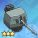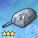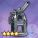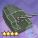# 127mm单装两用炮

2021-05-03更新

IJN_Hibiki

## 基础数据

• 127mm单装两用炮
• 伤害
• 初始 3×2
• 强化+6(满) 8×2
• 标准射速
• 初始 1.70s/轮
• 强化+6(满) 1.54s/轮
•  炮击 5
•  防空 7
• 弹药 通常弹
•  飞行速度 18
• 对甲比例 100-50-20
• 装备参数
•  索敌范围 60
•  索敌角度 360
• 弹药射程 40-60
•  散布角度 15
• 所属阵营 重樱
• 伤害修正比例
• 强化+7前 125%
• 强化+7 130%
• 伤害属性类型 炮击
• 属性效率 100%
• 适用舰种
驱逐
轻巡
重巡
超巡
战巡
战列
轻航
航母
航战
潜母
重炮
潜艇
维修
运输
风帆S
风帆V
风帆M

整装获取->->▷查看消耗

## 装备导航

鱼雷 • 星云舞裙  • 引力舞鞋  • 闪耀之歌  • 活力之歌  • 炽烈之歌  • 改良声呐  • 基础声呐  • 维修工具  • 陀螺仪  • 改良锅炉  • 灭火器  • 海军迷彩  • 液压舵机
舰炮 • 超重弹  • 珍珠之泪  • SG雷达  • 雪顶之梦  • 火控雷达  • 对空雷达
舰炮 • 海大黄蜂  • 海怒  • 海毒牙  • 海喷火  • 海飓风  • 海斗士 • 飞龙  • 火冠  • 火把  • 梭鱼  • 青花鱼  • 剑鱼 • 萤火虫  • 海燕  • 贼鸥 • 小王冠  • 归航信标 • 刺猬弹
舰炮 • 127mm单装两用炮 • 紫电改二  • 烈风 • 流星  • 天山改  • 天山 • 彗星 • Z旗  • 刺绣锦囊 • 晴岚  • 强风  • 瑞云
舰炮 • 梅之语  • 四神之印 • 上游-1
舰炮 • 海魂迷彩
舰炮 • 天使之羽
舰炮 • 飓风旗  • 帆索组件
设备 • 智慧模块  • 晃悠悠
设备 • 鮟鱇肝  • 玉米灯笼  • 组徽
设备 • 心之钥匙  • 觉醒宝珠
设备 • 偶像手环
设备 • 煌翼炎龙  • 征战巨坦 • 木龙雕塑
设备 • 形意口琴  • 泡云弹车  • 创世之槌  • Ｎ／Ａ  • 默示录  • 万灵药剂  • 涡旋风精  • 震耳雷球  • 结晶冰精  • 炙烈炎烧
设备 • 忍术卷轴  • 忍者装束
通常 • 苦无  • 短剑  • 权杖  • 猎弓  • 轻弩  • 指挥刀  • 骑枪  • 大剑  • 手弩  • 铁剑  • 单手锤  • 双剑 • 莱伯君  • 全开标枪  • 摇曳悠然 • 幻想之环  • 皮罗什基 • 光风霁月 • 魅力四射  • 不再哭泣 • 鸾凤流丽 • 一张字条 • 烤白薯  • 马卡龙  • 帕帕纳西  • 甜馅馒头  • 公主蛋糕 • 监禁程序  • 龙的饰品  • 演出棒  • 怪兽种子  • 戴拿飞翼 • 百合长老  • 百花盛开  • 幽兰爪  • 爱瑟尔剑  • 暗夜星矢  • 闪耀梦想 • 太刀・钢